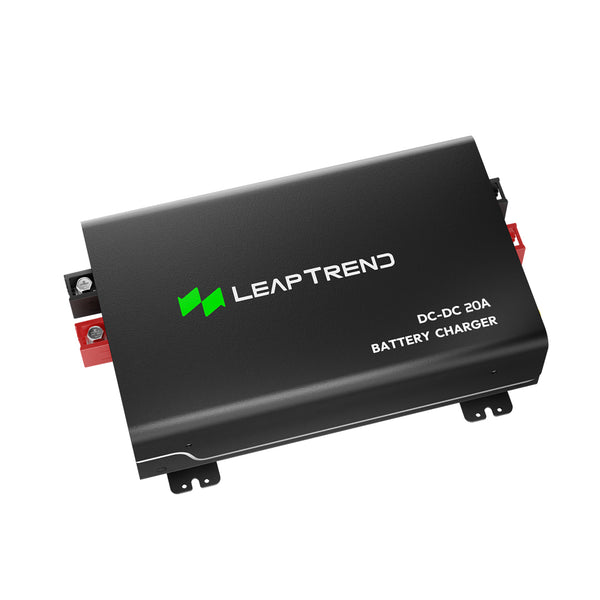# How Much Power Does a DC-DC Converter Consume?

Are you curious about the power consumption of a DC-DC converter? In this article, we will explore the factors that affect power consumption and provide insights into how much power a DC-DC converter typically consumes in different scenarios. Understanding this can help you make informed decisions when selecting and using DC converters for your electronic devices.

## Power Consumption in Idle Mode

When a DC-DC converter is in idle mode, meaning it is not actively converting power, its power consumption is relatively low. In this state, the converter draws a small amount of power to maintain its internal circuitry and standby functionality. Typically, the power consumption can range from a few milliwatts to a few watts, depending on the specific converter model and design.## Power Consumption under Light Load

Under light load conditions, where the converter is supplying power to a device that requires only a small amount of power, the power consumption remains relatively low. Modern DC power converters are designed to be highly efficient, with conversion efficiencies ranging from 80% to 95%. This means that the converter consumes only a fraction of the power it delivers to the load. For example, if the load requires 10 watts of power, the converter may consume around 11 to 12 watts, resulting in an efficiency of approximately 90%.

## Power Consumption under Heavy Load

When a DC is operating under heavy load conditions, supplying power to a device that requires a significant amount of power, its power consumption increases. The power consumption depends on various factors such as the input voltage, output voltage, and load current. Generally, the power consumption of a DC power converter under heavy load can range from a few watts to tens of watts. It is important to select a converter with a suitable power rating to ensure efficient power delivery and avoid overloading the converter.## Efficiency and Power Loss

It's worth noting that DC power converters are not 100% efficient, and a certain amount of power is lost during the conversion process. The efficiency of a converter is defined as the ratio of output power to input power. The remaining power is dissipated as heat. Higher efficiency converters minimize power loss and reduce the overall power consumption.

In summary, the power consumption of a power supply converter depends on its operating mode, load conditions, and efficiency. While idle and light load power consumption is relatively low, heavy load conditions can result in higher power consumption. Understanding these factors can help you select an appropriate DC-DC converter for your specific power requirements and optimize power efficiency in your electronic devices.